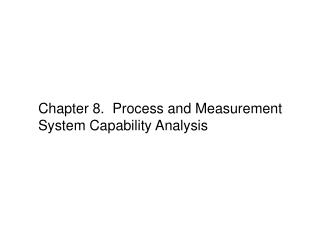# Chapter 8. Process and Measurement System Capability Analysis - PowerPoint PPT PresentationDownload PresentationChapter 8. Process and Measurement System Capability Analysis

Chapter 8. Process and Measurement System Capability AnalysisDownload Presentation## Chapter 8. Process and Measurement System Capability Analysis

- - - - - - - - - - - - - - - - - - - - - - - - - - - E N D - - - - - - - - - - - - - - - - - - - - - - - - - - -
##### Presentation Transcript

1. Natural tolerance limits are defined as follows: Process Capability

2. Uses of process capability

3. Process may have good potential capability Reasons for Poor Process Capability

4. Data collection steps:

5. Example 7-1, Glass Container Data

6. Mean and standard deviation could be estimated from the plot Probability Plotting • The distribution may not be normal; other types of probability plots can be useful in determining the appropriate distribution.

7. Process Capability Ratios Example 5-1:

8. Practical interpretation PCR = proportion of tolerance interval used by process For the hard bake process:

9. One-Sided PCR

10. Cp does not take process centering into account • It is a measure of potential capability, not actual capability

11. Measure of Actual Capability Cpk: one-sided PCR for specification limit nearest to process average

12. Normality and Process Capability Ratios • The assumption of normality is critical to the interpretation of these ratios. • For non-normal data, options are: • Transform non-normal data to normal. • Extend the usual definitions of PCRs to handle non-normal data. • Modify the definitions of PCRs for general families of distributions. • Confidence intervals are an important way to express the information in a PCR

13. Confidence interval is wide because the sample size is small

14. Confidence interval is wide because the sample size is small

15. Process Performance Index: Use only when the process is not in control.

16. Specifications are not needed to estimate parameters. Process Capability Analysis Using Control Chart

17. Since LSL = 200

18. Process Capability Analysis Using Designed Experiments

19. Gauge and Measurement System Capability Simple model: y: total observed measurement x: true value of measurement ε: measurement error

20. k = 5.15 → number of standard deviation between 95% tolerance interval Containing 99% of normal population. k = 6 → number of standard deviations between natural tolerance limit of normal population. For Example 7-7, USL = 60 and LSL = 5. With k =6 P/T ≤ 0.1 is taken as appropriate.

21. Signal to Noise Ration (SNR) SNR: number of distinct levels that can be reliably obtained from measurements. SNR should be ≥ 5 The gauge (with estimated SNR < 5) is not capable according to SNR criterion.

22. Discrimination Ratio DR > 4 for capable gauges. For example, 7-7: The gauge is capable according to DR criterion.

23. Usually conducted with a factorial experiment (p: number of randomly selected parts, o: number of randomly selected operators, n: number of times each operator measures each part) Gauge R&R Studies

24. This is a two-factor factorial experiment. • ANOVA methods are used to conduct the R&R analysis.

25. Negative estimates of a variance component would lead to filling a reduced model, such as, for example:

26. σ2: repeatability variance component → → For the example: With LSL = 18 and USL = 58: This gauge is not capable since P/T > 0.1.

27. Linear Combinations

28. Nonlinear Combinations → R is higher order (2 or higher) remainder of the expansion. R → 0 →

29. Assume I and R are centered at the nominal values. α = 0.0027: fraction of values falling outside the natural tolerance limits. Specification limits are equal to natural tolerance limits. Assuming V is approximately normal: →

30. For normal distribution with unknown mean and variance: Estimating Natural Tolerance Limits • Difference between tolerance limits and confidence limits • Nonparametric tolerance limits can also be calculated.